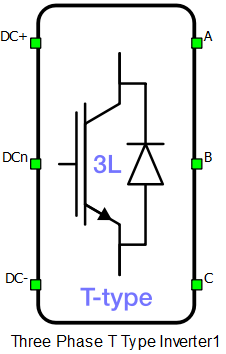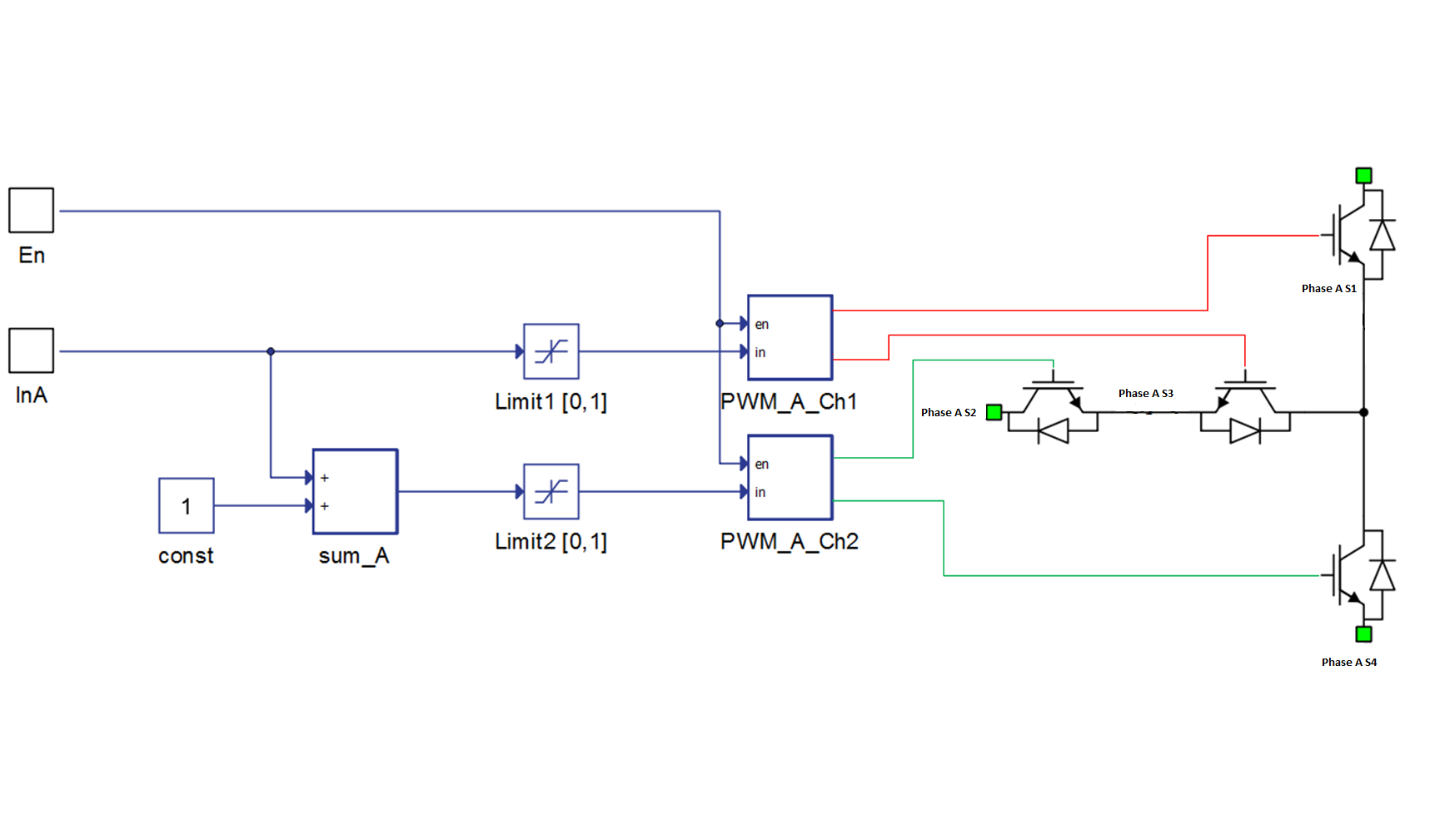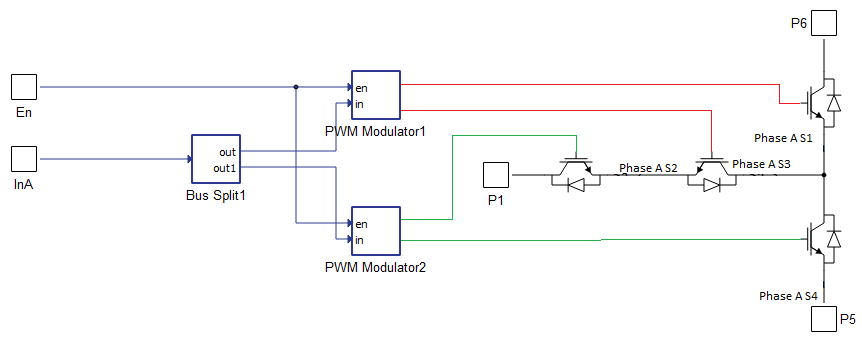# Three Phase T Type Inverter

Description of the Three-phase three-level T-type inverter/rectifier component in Schematic Editor Library

A block diagram and input parameters for a three phase, three level, T-type inverter-rectifier block are given in Table 1.

Table 1. A three-phase three-level inverter block in the HIL Toolbox
component component dialog window component properties• Property tabs
• General
• Timing
• Weight = 3

The schematic block diagram of the inverter switching block, with corresponding switch arrangement and naming, is given in Figure 1.

Selecting Digital inputs as the Control parameter enables assigning gate drive inputs to any of the digital input pins (from 1 to 32(64)). For example, if Phase A S1 is assigned to 1, the digital input pin 1 will be routed to the Phase A S1 switch gate drive. In addition, the gate_logic parameter selects either active high (High-level input voltage VIH turns on the switch), or active low (Low-level input voltage VIL turns on the switch) gate drive logic, depending on the external controller design.Figure 1. The schematic block diagram of a three-phase three-level T-type inverter/rectifier block diagram with corresponding switch naming

Selecting Internal modulator as the Control parameter, enables use of the internal PWM modulator for driving the switches instead of the digital input pins. In this configuration, four additional component inputs will be present. En input is used to enable/disable the internal PWM modulator, while InA, InB and InC are used as a reference signal inputs. Overall, 6 PWM channels are used to drive the three level three phase NPC T type converter, 2 per phase. Reference signals for the 2 modulators that control the switches of a single phase leg are created from a single reference input. The block diagram of a one phase leg controlled by PWM modulators is shown in Figure 2. The input reference signal for one modulator has a value range between -1.0 and 1.0. This signal is split into two reference signals for two modulators, and set in a range from 0.0 to 1.0.Figure 2. Block diagram of PWM control unit for NPC T-type phase legInternal modulator separated, when selected for Control parameter, enables the user to use internal PWM modulator for driving the converter's switches instead of digital input pins. Additional components inputs and number of created internal PWM modulators is the same as described in Internal modulator section. Reference signals for 2 modulators, that are controlling the switches of a single phase leg, are provided to the component through a vectorized input (the value on index 0 controls the PWM_A_Ch1 modulator, while the value on index 1 controls the PWM_A_Ch2 modulator). The block diagram of a one phase leg separately controlled by PWM modulators is shown in Figure 3. The permissible range for the input reference signals is adjustable from the component mask.

Model, when selected for Control parameter, enables the user to set the IGBTs' gate drive signals directly from signal processing model. The input pin 'gates' appears on the component and requires a vector input of twelve gate drive signals in the following order: [Phase A S1, Phase A S2,…, Phase B S1, Phase B S2,…, Phase C S1, Phase C S1,...]. When controlled from the model, logic is always active high.

Switching enabled, when checked, enables using an external PWM enabling digital signal.Figure 3. Block diagram of separated control method for NPC T-type phase leg

DTV detection, when enabled DTV detection will be signalized during simulation runtime.

## Timing

When Enable delays is enabled, turn on and turn off delay of the IGBTs will be included in the simulation. More information about this feature can be found on the dedicated section switching delay.

## Losses calculation

When the Losses calculation property is enabled, the component will calculate switching and conduction power losses for all switching elements (IGBTs and Diodes or MOSFETs). In the case of MOSFET switching elements, the diode characteristic represents the internal MOSFET body diode. Switching power losses are calculated as a function of current, voltage, and temperature using 3D lookup tables. Also, 2D input for losses is supported. When a 2D losses table is inserted, it assumes only current (I) and temperature dependence. From version 2020.3, conduction power losses can be defined as a function of current and temperature using Vt and Vd lookup tables (LUTs) (the previous option of using Vce, Rce, Vd, and Rd properties are removed). These LUTs can be 1D or 2D tables. If the LUT is a 1D table, forward voltage drop depends only on current, but if LUT is a 2D table, forward voltage drop dependence on the junction temperature is also considered. In the MOSFET case under reverse current conduction, a current sharing calculation between the MOSFET channel and the internal body diode is performed. Import options and an explanation how to correctly fill all necessary power losses parameters is described in the import power losses section.

In the case of IGBT switch type for both Losses groups, input/output terminals for power losses power losses receive/generate vectors of 24 elements (every index in the vector represent one switching element). The first 8 elements (indexes 0-7) are related to the switching elements in phase A, the second 8 elements (indexes 8-15) are related to the switching elements in phase B and the last 8 elements (indexes 16-23) are related to the switching elements in phase C. In the case of MOSFET switch type for both Losses groups, input/output terminals for power losses receive/generate vectors of 12 elements (every index in the vector represent one switching element). The first 4 elements (indexes 0-3) are related to the switching elements in phase A, the second 4 elements (indexes 4-7) are related to the switching elements in phase B, and the last 4 elements (indexes 8-11) are related to the switching elements in phase C. If different switch types are used for Losses groups, input/output terminals for power losses receive/generate vectors of 18 elements, with the same ordering logic as previously described. Switching element ordering and group definition for each phase are described in the T-Type Leg Losses calculation section. All switches are distributed in two groups, and for each group, different power loss parameters can be specified (Phase A S1, Phase A S4, Phase B S1, Phase B S4, Phase C S1, and Phase C S4 are in group 1, Phase A S2, Phase A S3, Phase B S2, Phase B S3, Phase C S2, and Phase C S3 are in group 2). Available Mask properties are:
• Losses groups - Switching elements group
• Current values - Switching elements current axis [A]

• Voltage values - Switching elements voltage axis [V]

• Temp values - Switching elements temperature axis [°C]

• Vce - IGBT collector emitter saturation voltage [V] - discontinued from 2020.3 release

• Rce - IGBT on state slope resistance [Ohm] - discontinued from 2020.3 release

• Vd - IGBT Diode voltage drop [V] - discontinued from 2020.3 release

• Rd - IGBT Diode slope resistance [Ohm] - discontinued from 2020.3 release

• Vt table - Switch forward voltage drop, f(I,T) [V]

• Vd table - Diode forward voltage drop, f(I,T) [V]

• Et on table - Switch switching ON losses, output energy, f(I, V, T) [J]

• Et off table - Switch switching OFF losses, output energy, f(I, V, T) [J]

• Ed off table - Diode switching OFF losses, output energy, f(I, V, T) [J]

Note: Automatic initialization of the Vt table and Vd table properties will be performed based on the discontinued Voltage and Resistance properties (Vce, Rce, Vd, Rd).

## Temperatures calculation

When Temperatures calculation property is enabled, component will calculate combined power losses (P_loss) and junction temperatures (T_junctions) for all switching elements (IGBTs and diodes). Combined power losses represent sum of the calculated switching and conduction losses transfered through internally generated Thermal network component. Internally generated Thermal network component also calculates junction temperatures from power losses, input cases temperatures and provided thermal model parameters. For each switching group different thermal parameters can be specified. Input/output ports for temperatures calculation are vectors of 24 elements and they are indexed in the same way as explained in the Losses calculation section. Additional temperatures calculation mask properties are:
• Thermal networks type - Defines type of internal thermal network
• Rth switch - List of thermal resistance for the IGBT switch
• Tth switch / Cth switch - List of thermal time constants or thermal capacitances for the IGBT switch
• Rth diode - List of thermal resistance for diode
• Tth diode / Cth diode - List of thermal time constants or thermal capacitances for diode
• Caculations execution rate - Execution rate in [s] for the losses and temperatures calculation logic

## Vienna rectifier optimization

Vienna rectifier optimization is activated through the corresponding checkbox in the Advanced tab. Sx_1 and Sx_4 IGBTs of each leg are removed, leaving only the diodes. This results in a topology commonly known as a Vienna rectifier. This reduces time slot utilization and can enable the model to run at a shorter simulation time step.

## Digital Alias

If a converter is controlled by digital inputs, an alias for every digital input used by the converter will be created. Digital input aliases will be available under the Digital inputs list alongside existing Digital input signals. The alias will be shown as Converter_name.Switch_name, where Converter_name is name of the converter component and Switch_name is name of the controllable switch in the converter.